Precalculus : Prove Trigonometric Identities

Example Questions

Example Question #1 : Prove Trigonometric Identities

Simplify: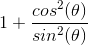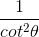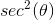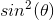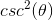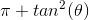Explanation:

To simplify, find the common denominator and multiply the numerator accordingly.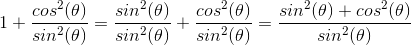The numerator is an identity.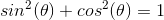Substitute the identity and simplify.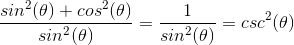Example Question #2 : Prove Trigonometric Identities

Evaluate in terms of sines and cosines: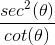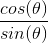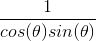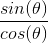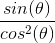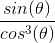Explanation:

Convertinto its sines and cosines.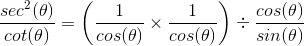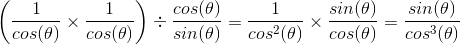Example Question #3 : Prove Trigonometric Identities

Please choose the best answer from the following choices.

Simplify the following: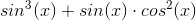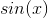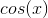Already in simplified form.Explanation:First factor out sine x.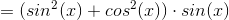Notice that a Pythagorean Identity is present.

The identity needed for this problem is: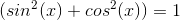Using this identity the equation becomes,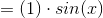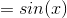.

All Precalculus Resources